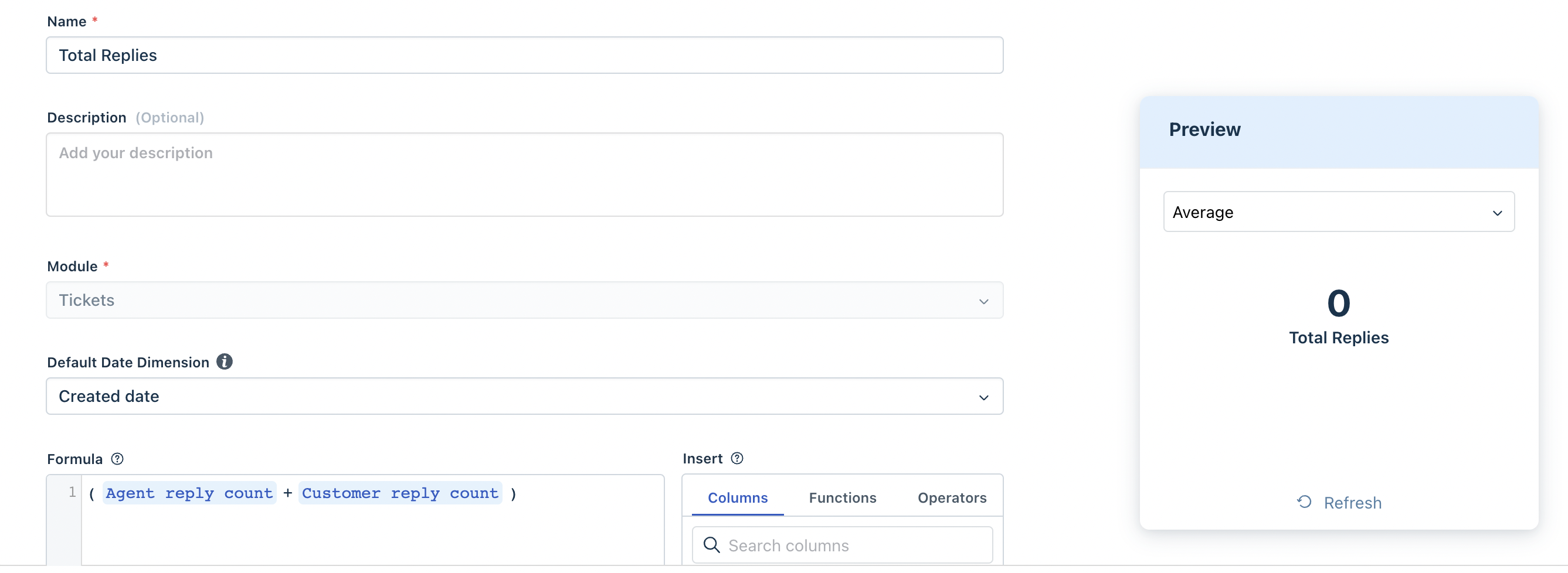Freshdesk Analytics has an extensive list of general helpdesk metrics as default, and custom metrics are here to create a much more curated experience for your organization. As an enterprise business, you can now create custom metrics using single arithmetic operations or even complex functions to stay on top of the metrics most relevant to your business.

Here is a brief guide to all the functions and operators you can use to get started and build your own metrics:

## What is a metric?

A metric is a number property that you can measure or operate on.

Example: Tickets reopened is a metric that measures the number of times a ticket was reopened

## How to create custom metrics?

• Goto Analytics tab from your left pane and click on the Settings icon on the top-right corner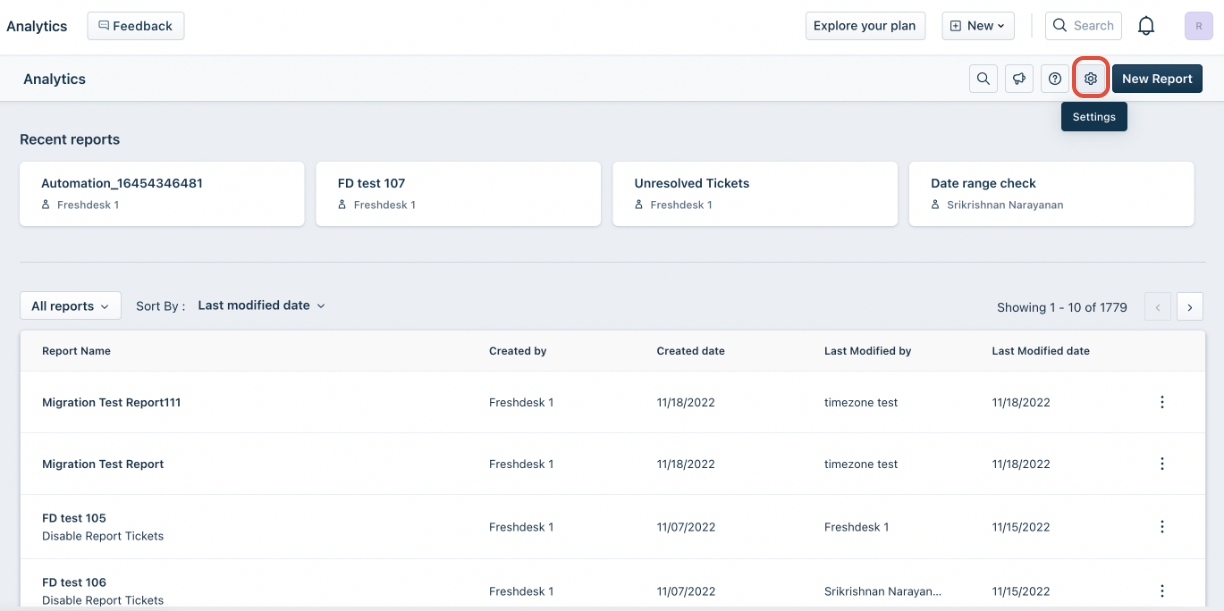• Select “Custom Metrics” from the side menu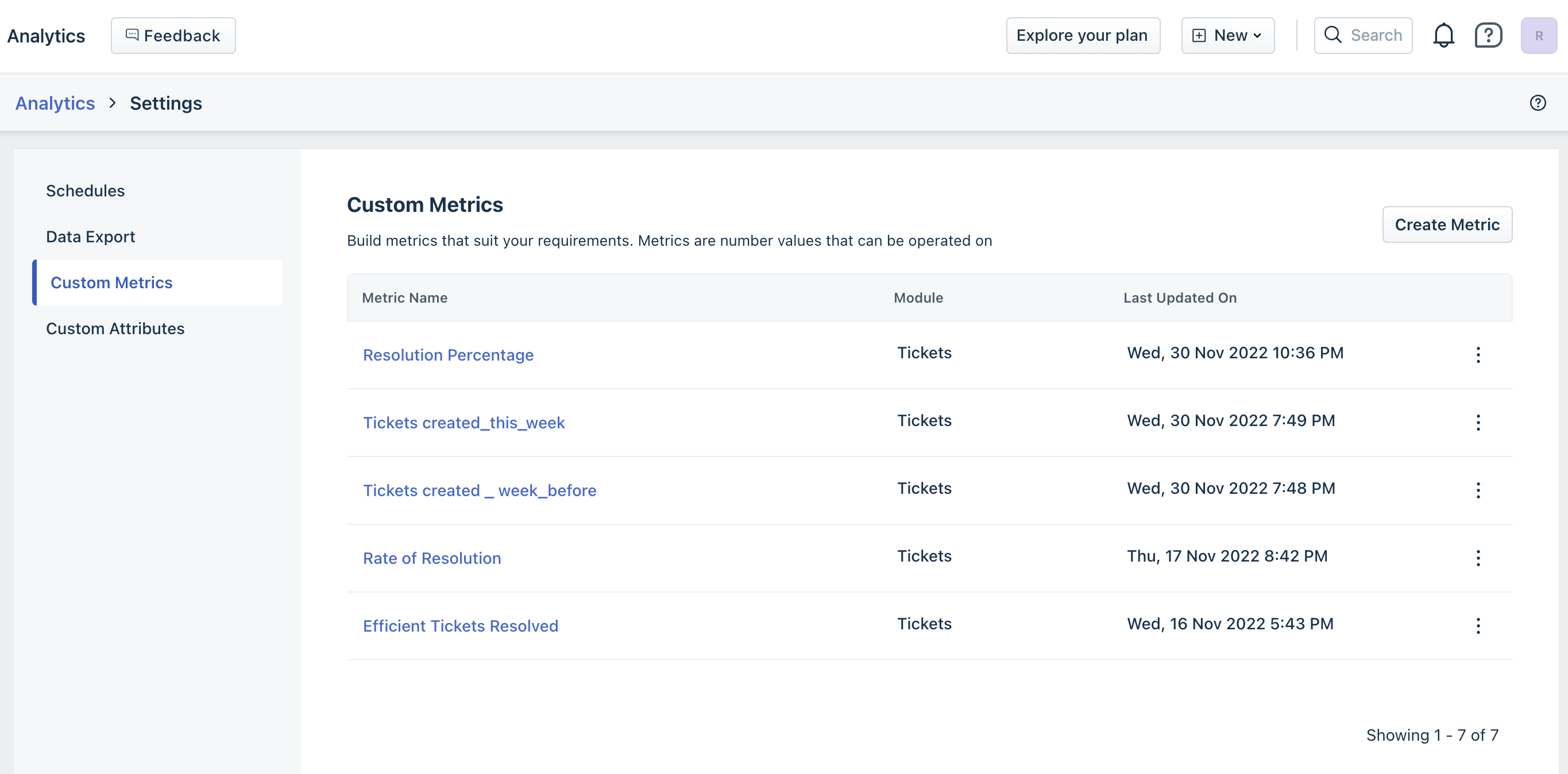• Click on “Create Metric” to start creating your custom metrics by using a combination of functions or operators.
• Once you come up with your formula, you can test it by clicking on 'Check syntax'. If your formula is correct, there will be a green 'Valid' that will appear to the left of the 'Check syntax' button. You can also see a preview of your result in the 'Preview' window.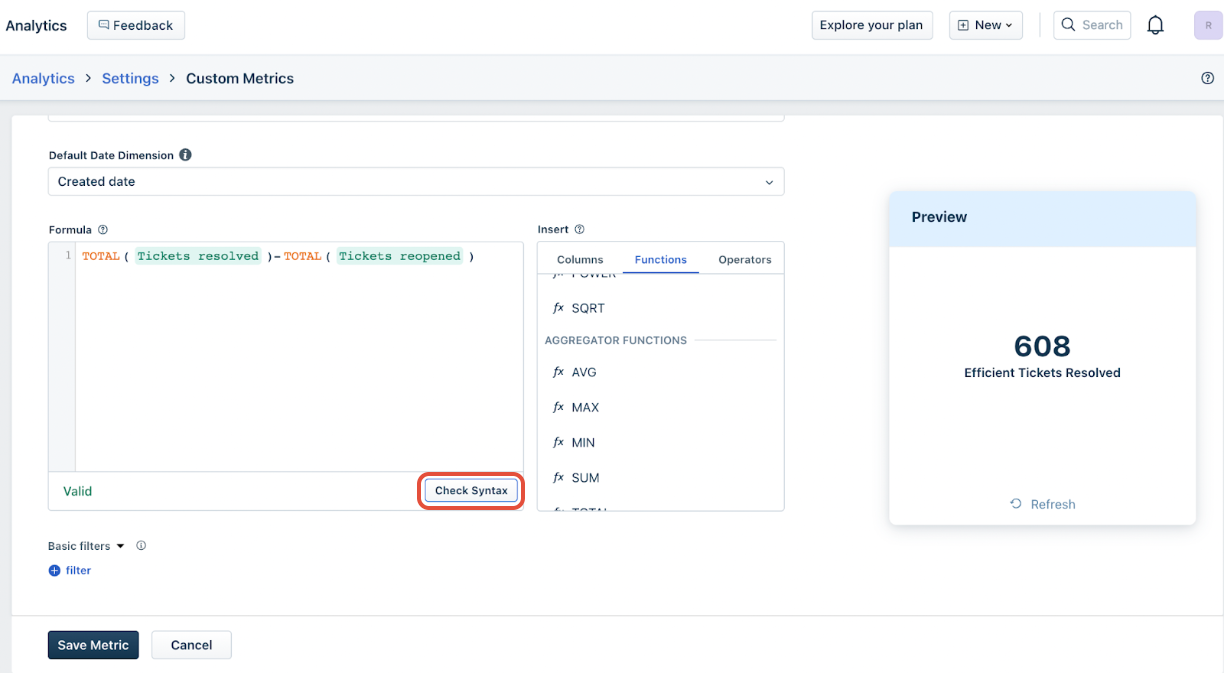• Click “Save Metric” once done
• Use this custom metric for creating widgets or wherever required

Note: Only users with edit and export access can create and modify the custom metrics.

## Custom metrics functions:

You can use all the functions shown below to create and customize your existing metrics.

 COMMAND MEANING Function - Number fx CEIL Returns the least integer greater than or equal to X Example CEIL (2.5) is 3 fx FLOOR Returns the largest integer lesser than or equal to X Example FLOOR (2.5) is 2 fx ROUND Round the number up to nearest integer value. Example ROUND (2.5) is 3 fx EXP Returns the value of e (Euler's number) to the power of X Example EXP (2) is 7.38906 fx GREATEST Returns greatest value among all fields Example GREATEST (1, 2, 3) returns 3 fx LEAST Returns least value among all fields Example LEAST (1, 2, 3) returns 1 fx LN Returns the value of the natural logarithm function of X (base e logarithm of X) Example LN (2) is 0.6931471805599453 fx POWER Returns the value of X to the power of Y Example POWER (2, 3) will return 8 fx SQRT Returns the value of the square root of X. Example SQRT (4) will return 2 Function - Aggregator fx AVG Returns the average value of a numeric expression X Example AVG ( Number ) fx MAX Returns the maximum value of a numeric expression X Example MAX ( Number ) fx MIN Returns the minimum value of a numeric expression X Example MIN ( Number ) fx SUM Returns the sum value of a numeric expression X Example SUM ( Number ) Function - Operators + Addition - Subtraction * Multiplication / Division % Modular Division

## How to access the metrics you create?

• Go to the Analytics Tab and select your report.
• Click on edit in the top right corner
• Search for the metric from the right pane. Choose the respective metric from the dropdown and click on Apply and add it to the widget.## Sample Metrics:

1. Measuring efficient tickets resolved by calculating and subtracting them from reopened tickets.
• Formula: Total(Tickets resolved) - total(tickets reopened)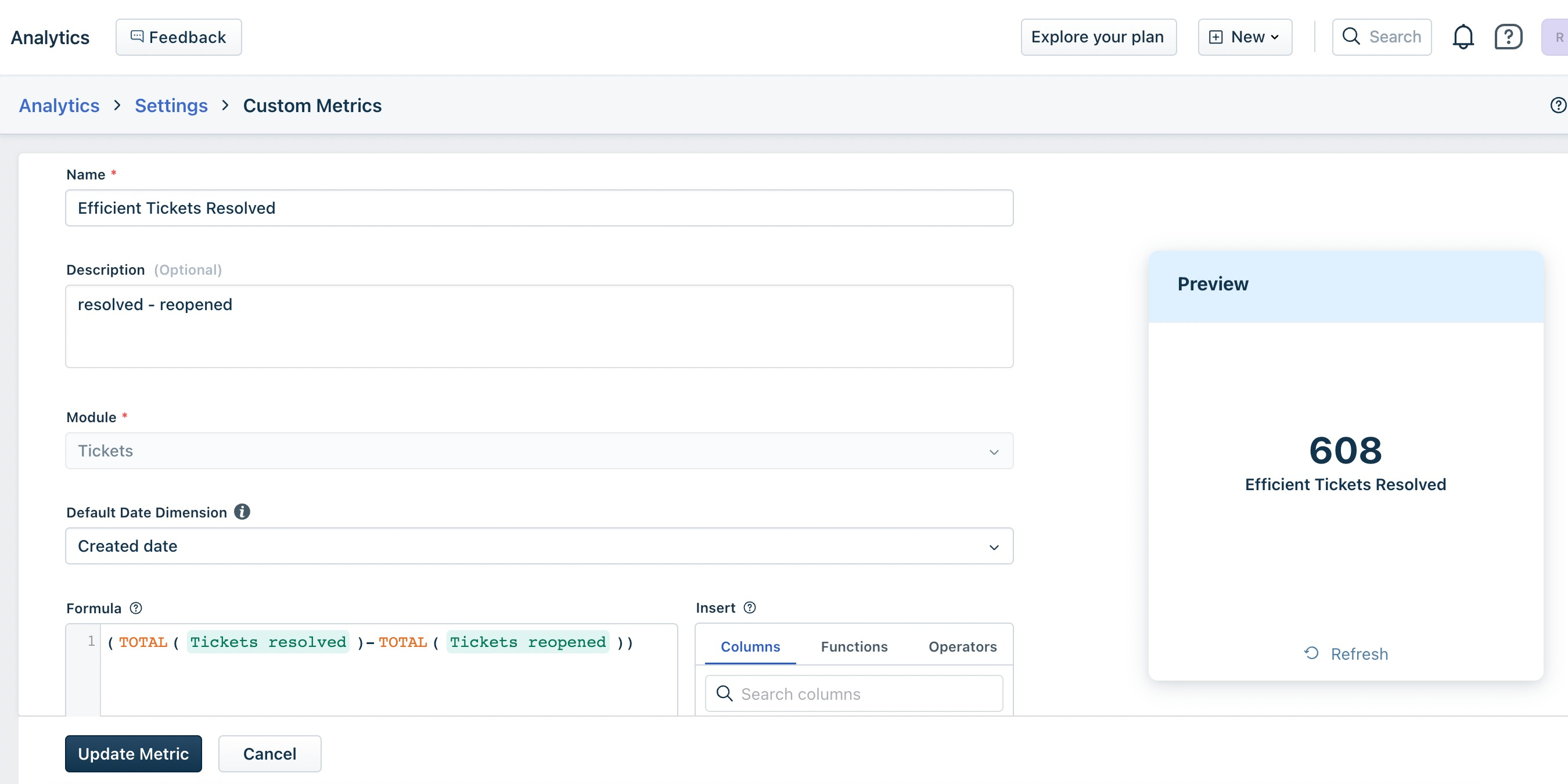2. Creating a metric called actual resolution time measures the time taken to resolve a ticket after it is in ‘X’ status to the time it was resolved.
• Formula: Sum(Time spent in business hours)
• Filter: list of statuses3. Measuring the number of emails and not the number of customer interactions. Therefore the first email customer sends is the first email.
• Formula: Total(customer interactions) + 1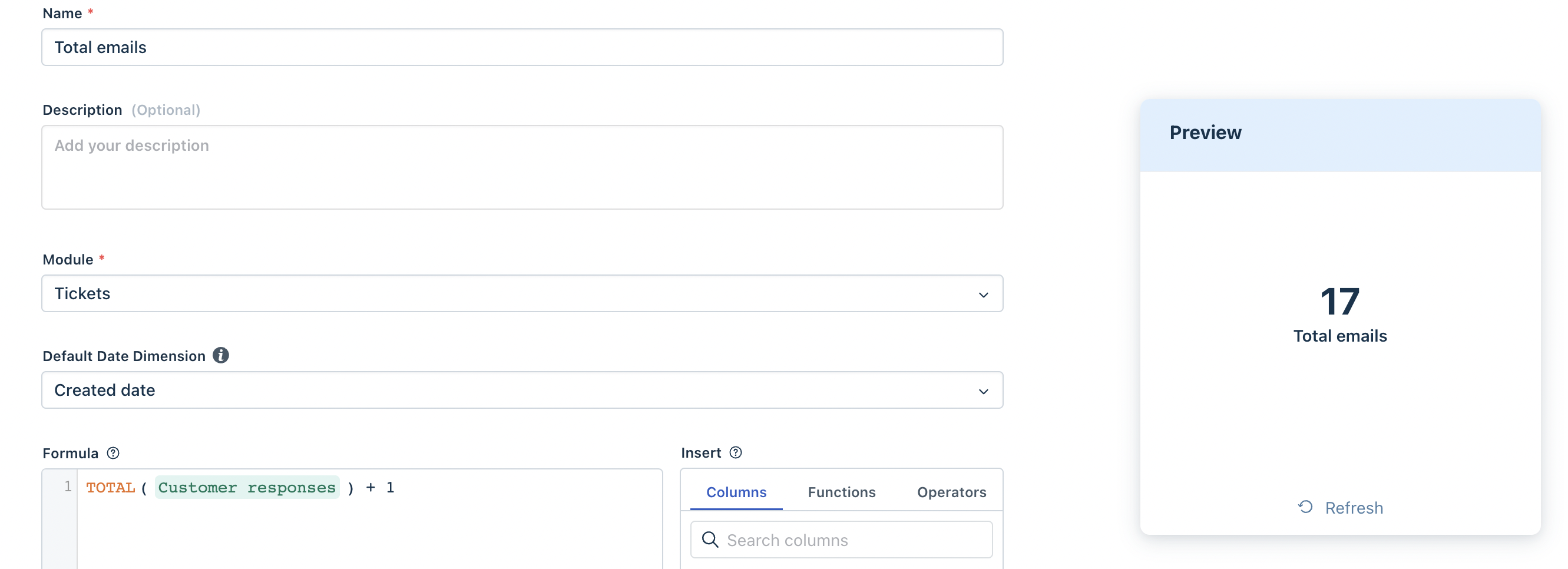4. Track a metric like “Total Replies,” which would be a combination of both “Agent Replies” and “Customer Replies”.
• Formula: Total Replies = Agent reply count + Customer Reply count